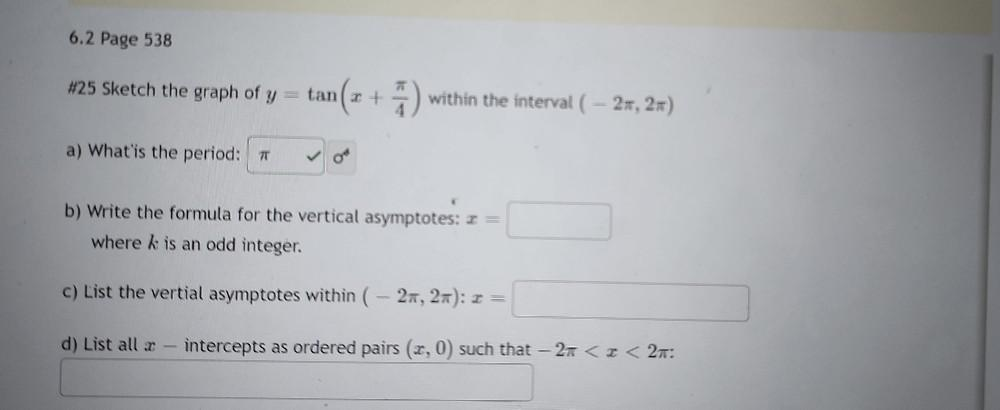Question:

# Sketch the graph of y = tan( x + π/4) within the interval ( – 2π, 2π) a) What is the period? b) Write the formula for the vertical asymptotes: x = where k is an odd integer, c) List the vertical asymSketch the graph of y = tan( x + π/4) within the interval ( – 2π, 2π) a) What is the period? b) Write the formula for the vertical asymptotes: x = where k is an odd integer, c) List the vertical asymptotes within ( – 2π, 2π): x = d) List all x intercepts as ordered pairs (x, 0) such that – 2π < x < 2π: